# Probabilistic Programming 4: Linear Gaussian Dynamical System¶

## Monte Carlo sampling¶

### Preliminaries¶

• Goal
• Learn to apply Turing to a dynamical system.
• Materials
• Mandatory
• These lecture notes.
• Optional
In [ ]:
# Package managing
using Pkg
Pkg.activate("workspace")
Pkg.instantiate();

using Logging; disable_logging(LogLevel(0))
using Distributions
using StatsPlots
using Turing
using MCMCChains
include("../scripts/pp-4.jl");


## Data generation¶

In this notebook, we will be considering continuous state-space models. We will focus on the simplest form: the Gaussian dynamical system:

\begin{align} x_k =&\ A x_{k-1} + q_{k-1} \\ y_k =&\ H x_k + r_k \, , \end{align}

where $x_k \in \mathbb{R}^{N}$ is the state, $y_k \in \mathbb{R}^{M}$ is the measurement. Process noise and measurement noise are assumed to be zero mean Gaussian with covariance matrices $Q$ and $R$ respectively.

We'll generate the data in the same manner as the HMM setting. We start with an initial state $x_0$, apply the state transition $Ax_0$, and add Gaussian noise $q_0$. We then take the current state $x_1$, apply the emission transformation $H x_1$ and add Gaussian noise again $r_1$. This is repeated until we've reached $T$ observations.

In :
# Dimensionalities
N = 2
M = 2

# Length of time-series
T = 50

# Lenght of time step
Δt = 1.0

# Transition matrix of latent variables
transition = [1.0 Δt;
0.0 1.0]

# Emission matrix for observed variables
emission = [1.0 0.;0. 1.0]

# Process noise (latent variables)
process_noise = [0.2*Δt 0.;0. 0.1*Δt]

# Measurement noise (observations)
measurement_noise = [0.1 0.;0. 0.1]

# Preallocate data arrays
states = zeros(2,T)
observations = zeros(2,T)

# Initial state
state_0 = [0., 0.]

# Keep previous state in memory
state_tmin = state_0

# Generate data for entire time-series
for t = 1:T

# Transition from previous state
states[:,t] = rand(MvNormal(transition * state_tmin, process_noise), 1)[:,1]

# Emission of current state
observations[:,t] = rand(MvNormal(emission * states[:,t], measurement_noise), 1)[:,1]

# Update previous state
state_tmin = states[:,t]

end

# Visualization of states
plot(states[1,:], states[2,:], color="red", label="states", grid=false, xlabel="position x-coordinate", ylabel="position y-coordinate")
scatter!(observations[1,:], observations[2,:], color="blue", label="observations")

Out: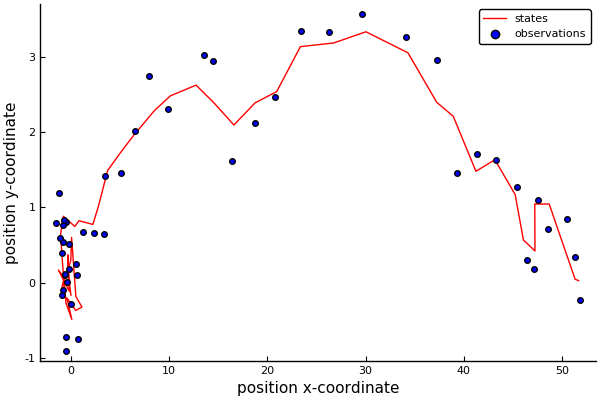Since we don't have a time-axis anymore, it is hard to see how the process evolves. Animating the path is helpful in this regard.

In :
"Animation of cart's path"

# Plot initial state
plot([states[1,1]], [states[2,1]],
color="red",
label="states",
grid=false,
xlabel="position x-coordinate",
ylabel="position y-coordinate",
xlims=[-60,60],
ylims=[-10,10])

# Plot initial observation
scatter!([observations[1,1]], [observations[2,1]], color="blue", label="observations")

anim = @animate for t = 2:T

title!("t = "*string(t))

# Plot new state
plot!([states[1,t-1:t]], [states[2,t-1:t]], color="red", label="")

# Plot new observation
scatter!([observations[1,t]], [observations[2,t]], color="blue", label="")

end

gif(anim, "visualizations/example-GDS.gif", fps = 3)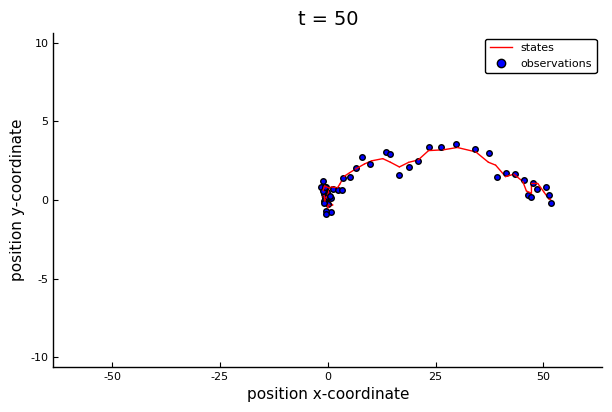Out: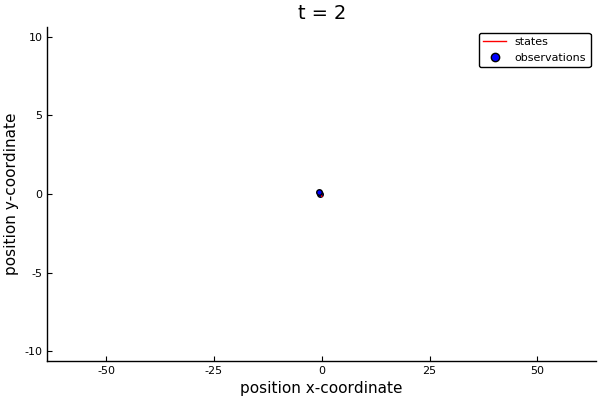## Model specification¶

The Bayesian formulation of the LGDS state-space model takes the following form:

\begin{align} p(x_k \mid x_{k-1}) =&\ \mathcal{N}(x_k \mid A x_{k-1}, Q)\\ p(y_k \mid x_k) =&\ \mathcal{N}(y_k \mid H x_k, R) \, . \end{align}

We can easily specify the states and observations as multivariate Gaussian distributions. If we do not know the process and measurement noise matrices, we can add Wishart priors to them. Similarly, if we don't know the transition and emission matrices, we can priors for them as well. Since these can take on negative values, we would specify them as multivariate Gaussians;

\begin{align} p(Q) \sim&\ \mathcal{W}(V_Q, n_Q) \\ p(R) \sim&\ \mathcal{W}(V_R, n_R) \\ p(A) \sim&\ \mathcal{N}(m_A, S_A) \\ p(H) \sim&\ \mathcal{N}(m_H, S_H) \, . \end{align}

These are all known distributions to sample from, and they are locally conjugate. However, a Gibbs sampler can be extremely slow in a time-series setting. Plus, with some component samplers, such as Particle Gibss, errors can propogate forward through the time-series, resulting in drifting estimates. We will run an agnostic MCMC procedure in this situation.

Again, we start simple and slowly improve the model.

### Model 1: estimate states¶

We will first assume we know the transition and emission matrices, as well as measurement and process noises. Based on these, we purely want to estimate states from observations.

In :
@model LGDS(y, transition, process_noise, emission, measurement_noise) = begin
"This model implicitly assumes 2-dim states and 2-dim observations"

# Time-series length
T = size(y, 2)

# State sequence.
x = Vector{Vector}(undef, T)

# Define initial state
x_0 ~ MvNormal([0., 0.], [1. 0.;0. 1.])

# Initialize previous state
x_tmin = x_0

# Loop over entire sequence
for t = 1:T

# State transition
x[t] ~ MvNormal(transition * x_tmin, process_noise)

# Observation emission
y[:,t] ~ MvNormal(emission * x[t], measurement_noise)

# Update previous state
x_tmin = x[t]
end
end

# Call instance of the model
model1 = LGDS(observations, transition, process_noise, emission, measurement_noise);


We will use a "No U-Turn Sampler". Its parameters are the number of samples to "burn" and the acceptance ratio of the walk.

In :
# NUTS logger is cumbersome
Turing.turnprogress(false)

# Length of chain
len_chain = 1000

# Define sampler
sampler1 = NUTS(200, 0.6)

# Call sampler
chain1 = sample(model1, sampler1, len_chain);


Let's look at the final state of the chain.

In :
plot(chain1[Symbol("x["*string(T)*"]")])

Out: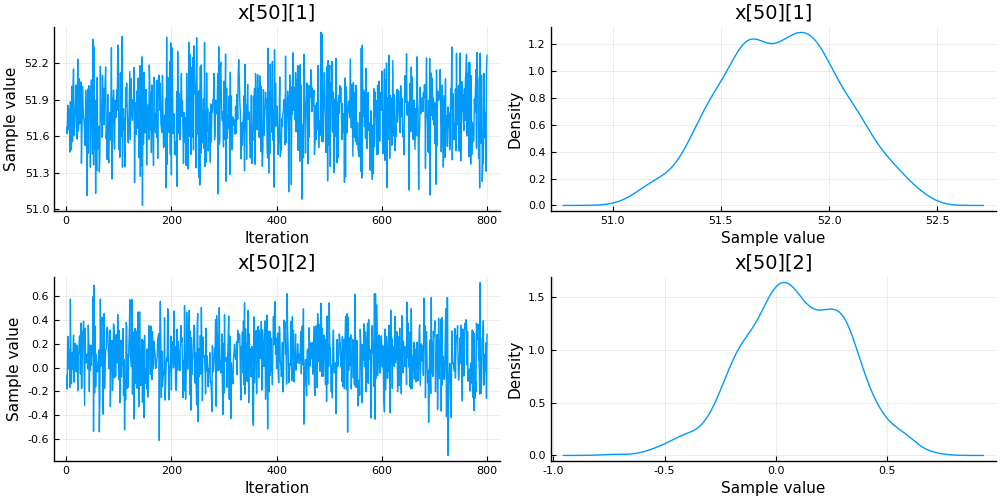And plot the inferred states.

In :
# Extract mean of the chain
x_hat = reshape(mean(chain1[:x].value.data, dims=), (2,50))

# Extract standard error of the mean
x_sem = reshape(std(chain1[:x].value.data, dims=), (2,50))
x_sem[1,:] ./= sqrt.(range(1, stop=T))
x_sem[2,:] ./= sqrt.(range(1, stop=T));

# Visualization
plot(states[1,:], states[2,:], color="red", label="states", grid=false)
plot!(x_hat[1,:], x_hat[2,:], ribbon=[x_sem, x_sem], fillalpha=0.2, color="green", label="inferred")
scatter!(observations[1,:], observations[2,:], color="blue", label="observations")

Out: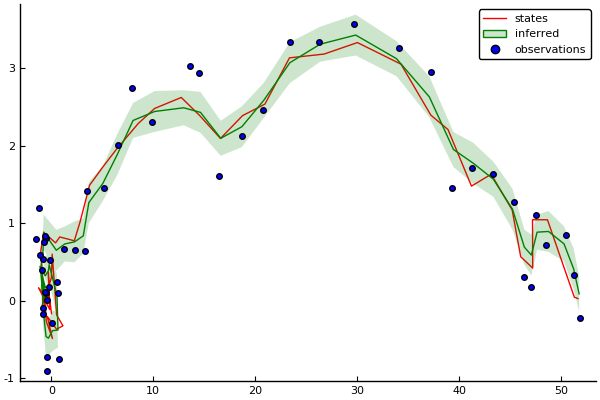### Model 2: process noise estimation¶

We can extend the model to include process noise estimation.

In :
@model LGDS(y, transition, emission, measurement_noise) = begin
"This model implicitly assumes 2-dim states and 2-dim observations"

# Time-series length
T = size(y, 2)

# Define prior for process noise
Q ~ Wishart(2, [1. 0.;0. 1.])

# State sequence.
x = Vector{Vector}(undef, T)

# Define initial state
x_0 ~ MvNormal([0., 0.], [1. 0.;0. 1.])

# Initialize previous state
x_tmin = x_0

# Loop over entire sequence
for t = 1:T

# State transition
x[t] ~ MvNormal(transition * x_tmin, Q)

# Observation emission
y[:,t] ~ MvNormal(emission * x[t], measurement_noise)

# Update previous state
x_tmin = x[t]
end
end

# Call instance of the model
model2 = LGDS(observations, transition, emission, measurement_noise);


Stick to the NUTS sampler.

In :
# Length of chain
len_chain = 1000

# Define sampler
sampler2 = NUTS(200, 0.6)

# Call sampler
chain2 = sample(model2, sampler2, len_chain);


Check the final state to see if chain has nicely converged.

In :
plot(chain2[Symbol("x["*string(T)*"]")])

Out: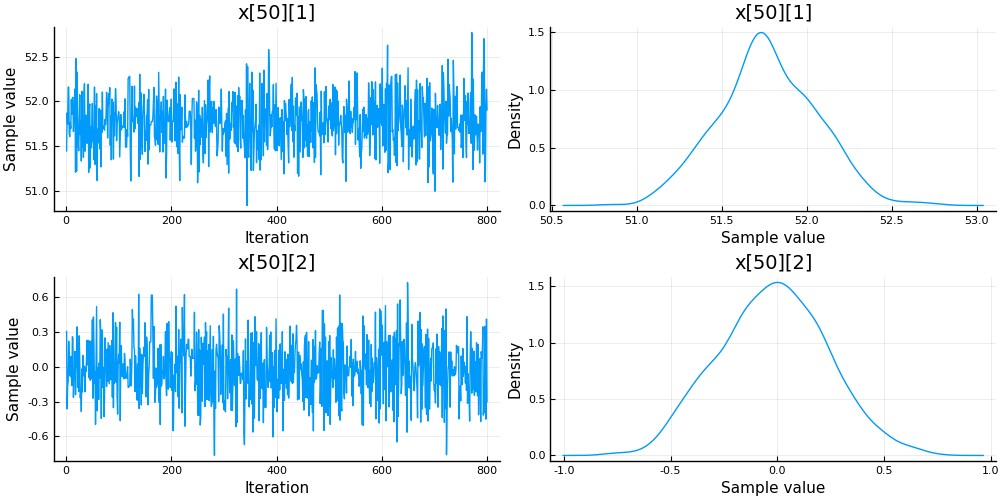Let's look at the state estimates. we expect them to be different from the previous run, because process noise is being changed during inference.

In :
# Extract mean of the chain
x_hat = reshape(mean(chain2[:x].value.data, dims=), (2,50))

# Extract standard error of the mean
x_sem = reshape(std(chain2[:x].value.data, dims=), (2,50))
x_sem[1,:] ./= sqrt.(range(1, stop=T))
x_sem[2,:] ./= sqrt.(range(1, stop=T));

# Visualization
plot(states[1,:], states[2,:], color="red", label="states", grid=false)
plot!(x_hat[1,:], x_hat[2,:], ribbon=[x_sem, x_sem], fillalpha=0.2, color="green", label="inferred")
scatter!(observations[1,:], observations[2,:], color="blue", label="observations")

Out: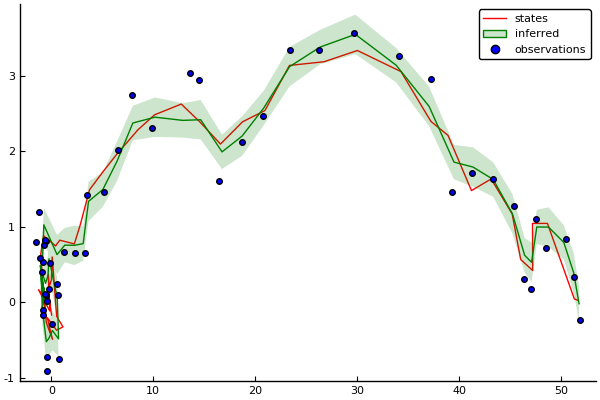Let's also have a look at the estimated process noise matrix.

In :
plot(chain2[:Q])

Out: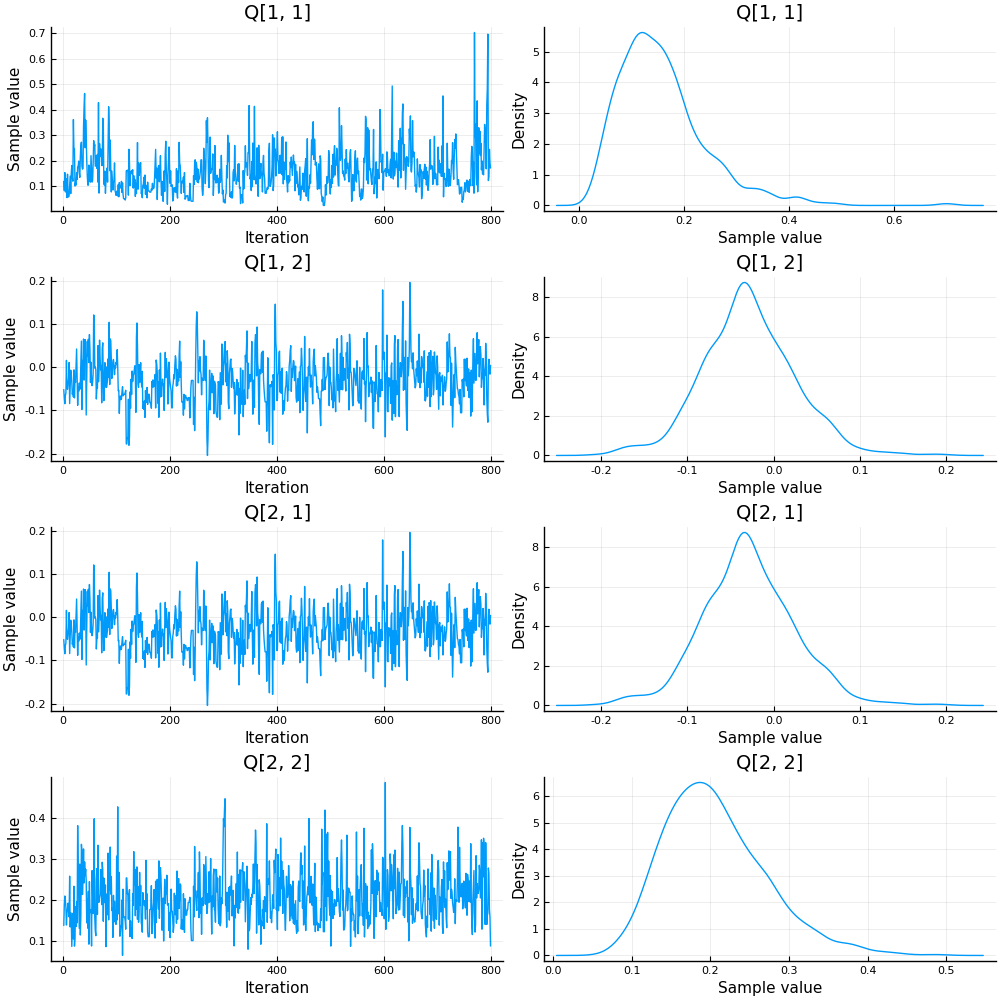We'll use a bar plot to visualize the coefficients.

In :
# Extract state estimates
pnoise_estimate = reshape(mean(chain2[:Q].value.data, dims=), (2,2))

# Visualization
l = @layout [a b]
h1 = bar(0:4, reshape(process_noise, (4,)), label="true", ylims=[0., 1.])
h2 = bar(reshape(pnoise_estimate, (4,)), label="estimated", ylims=[0., 1.])
plot(h1, h2, layout=l, size=(1000,500))

Out: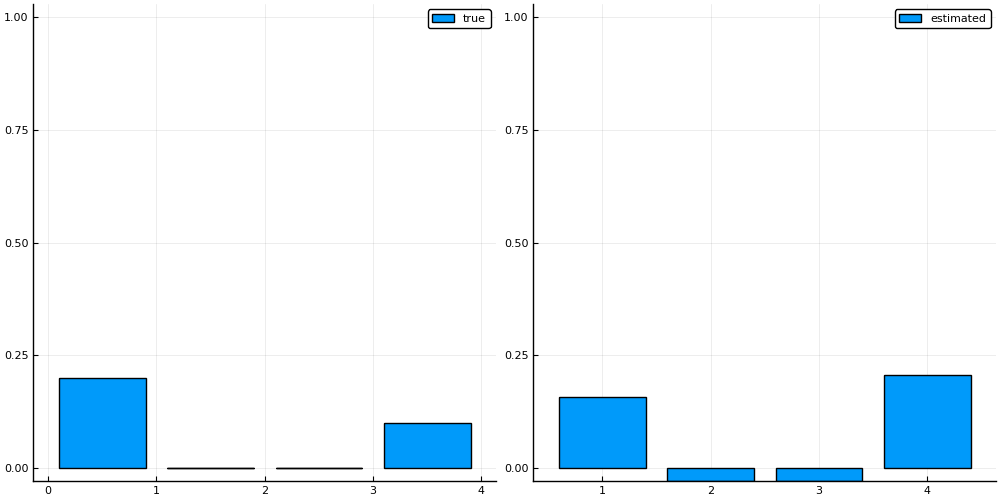### Optional assignment: transition matrix estimation¶

The transition matrix can be estimated in a similar fashion as the process noise matrix, except that it does not need to be positive semi-definite. Can you construct a model with states, process noise and the transition matrix as free variables?

In :
### Your code here